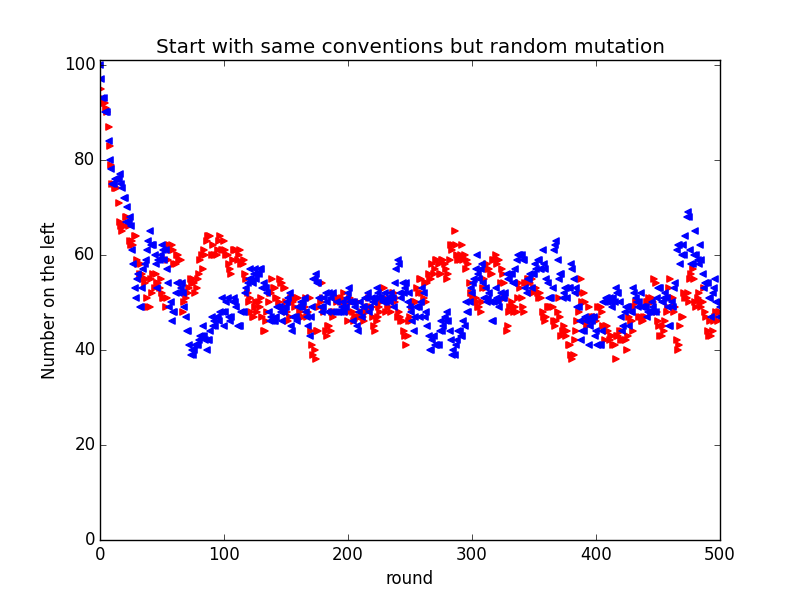### Evolutionary Mathematics [@drvinceknight](https://twitter.com/drvinceknight) {[github.com](https://github.com/Axelrod-Python/Axelrod), [gitter.im](https://gitter.im/Axelrod-Python/Axelrod)}/Axelrod-Python/Axelrod

## Themes

reveal.js comes with a few themes built in:
Black (default) - White - League - Sky - Beige - Simple
Serif - Night - Moon - Solarized

## Advice for foreigners on how Britons walk

“Telling people how to walk is simply not British.”
“But on the street? No, we don’t walk on the left or the right. We are British and wander where we will..”

import random

def walk(number_of_walks):
"""
Simulate people walking along the pavement
"""
reds = [random.choice('LR') for k in range(number_of_walks)]
blues = [random.choice('LR') for k in range(number_of_walks)]
bumps = sum([reds[k] != blues[k] for k in range(number_of_walks)])
return bumps


from bashplotlib.histogram import plot_hist  # cli plots

def plot_experiment(data):
return plot_hist(data,
height=20,  # Set the height
colour='red',  # Set the colour
bincount=20,  # Number of bins
showSummary=True)  # Show a summary

plot_experiment([walk(500) for k in range(1000)])


# Evolutionary dynamics with a mutation rate:
size_of_population = 100  # Number of people
number_of_rounds = 500  # How many rounds
mutation_rate = .05  # Chance of changing strategy
death_rate = .05  # Chance of removal

reds = ['L' for k in range(size_of_population)]
blues = ['L' for k in range(size_of_population)]
red_data = [sum([k == 'L' for k in reds])]
blue_data = [sum([k == 'L' for k in reds])]

for rnd in range(number_of_rounds):  # Loop through rounds
for j, pair in enumerate(zip(reds, blues)):  # Loop through players

if random.random() < mutation_rate:  # Check if random change
reds[j], blues[j] = random.choice('LR'), random.choice('LR')

if pair != pair:  # If bump
if random.random() < death_rate:  # If mind change
reds[j], blues[j] =  blues[j], reds[j]

red_data.append(sum([k == 'L' for k in reds]))  # Data collection
blue_data.append(sum([k == 'L' for k in blues]))### Prisoner's Dilemma

$$\begin{pmatrix} 3,3&0,5\\ 5,0&1,1 \end{pmatrix}$$
1. Robert Axelrod
2. 1980a: 14+1 strategies
3. 1980b: 64+1 strategies

TODO: Sacrifice a catclass TitForTat(Player):
"""A player starts by cooperating and then mimics previous move by opponent."""

name = 'Tit For Tat'
classifier = {
'memory_depth': 1,  # Four-Vector = (1.,0.,1.,0.)
'stochastic': False,
'inspects_source': False,
'manipulates_source': False,
'manipulates_state': False
}

@staticmethod
def strategy(opponent):
return 'D' if opponent.history[-1:] == ['D'] else 'C'


class TestTitForTat(TestPlayer):

name = "Tit For Tat"
player = axelrod.TitForTat
expected_classifier = {
'memory_depth': 1,
'stochastic': False,
'inspects_source': False,
'manipulates_source': False,
'manipulates_state': False
}

def test_strategy(self):
"""Starts by cooperating."""
self.first_play_test(C)

def test_effect_of_strategy(self):
"""Repeats last action of opponent history."""
self.markov_test([C, D, C, D])
self.responses_test([C] * 4, [C, C, C, C], [C])
self.responses_test([C] * 5, [C, C, C, C, D], [D])class MindBender(MindWarper):
"""
A player that changes the opponent's strategy by modifying the internal
dictionary.
"""

name = 'Mind Bender'
classifier = {
'memory_depth': -10,
'stochastic': False,
'inspects_source': False,
'manipulates_source': True,  # changes what opponent will do
'manipulates_state': False
}

@staticmethod
def strategy(opponent):
opponent.__dict__['strategy'] = lambda opponent: 'C'
return 'D'

pip install axelrod {[github.com](https://github.com/Axelrod-Python/Axelrod), [gitter.im](https://gitter.im/Axelrod-Python/Axelrod)}/Axelrod-Python/Axelrod [Blog posts by TC](http://www.thomascampbell.me.uk/category/axelrod.html) [demo.ipynb](https://github.com/drvinceknight/Talks/blob/gh-pages/2015-10-12-Evolutionary-mathematics/demo.ipynb)